Home > ACC7 > Chapter cc38 > Lesson cc38.3.1 > Problem8-130

8-130.
1. Find the value of each indicated angle in the following figures. Do not use a protractor. Use the properties of straight and vertical angles to help you. Homework Help ✎

2.  a.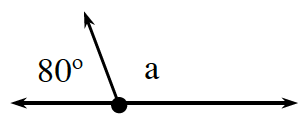b.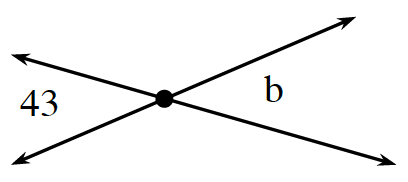c.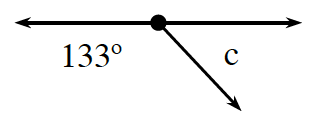d.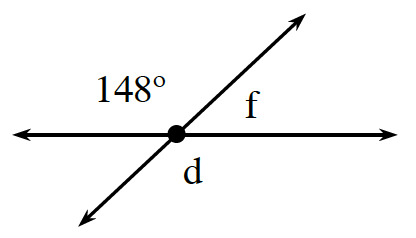e.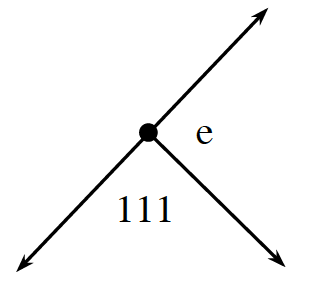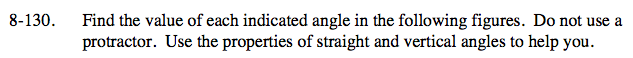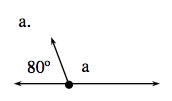These angles are supplementary. 180° - 80° = a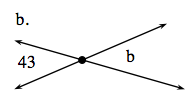43° and b are vertical angles. What does that tell you about the measure of angle b?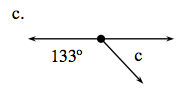Set up an equation relating 133° and d.
133° + c = 180°.

Subtract 133° from both sides.
133° + c = 180°.
−133° −133°
c = 47°

47°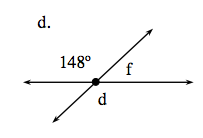d and 148° are vertical angles, and f and 148° are supplementary.

d = 148°: f = 32°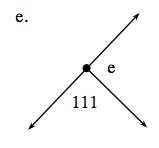111° + e = 180°. Refer to part (c) for the steps to solving this problem.